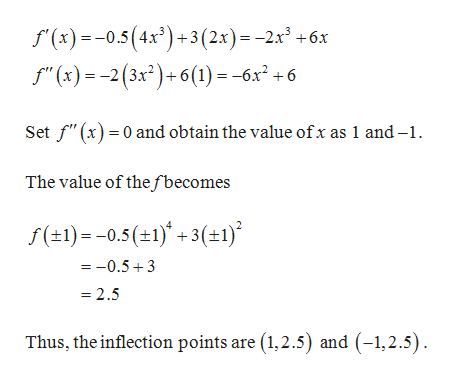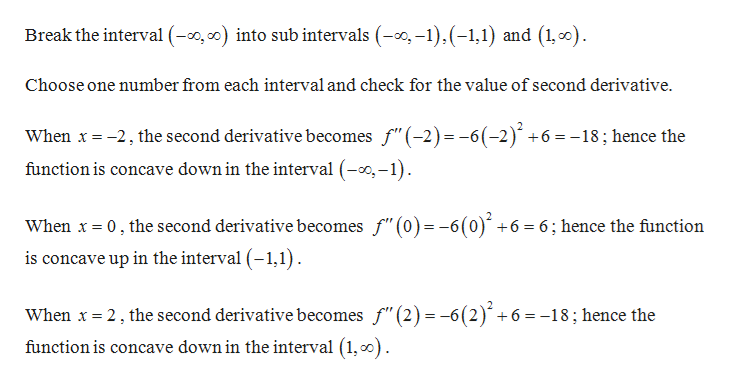f(x)=−0.5⋅x^4+3x^2 what are the interverls for concavity

Question

f(x)=−0.5⋅x^4+3x^2 what are the interverls for concavity

Step 1

Given:

Step 2

Obtain the second derivative in order to find the inflection point.help_outlineImage Transcriptionclose(x)0.5(4x)+3(2x) -2x +6x f (x)2 (3x2)+6(1)- -6x +6 Set f" (x)0 and obtain the value ofx as 1 and-1 The value of the fbecomes f(1)-0.5(1)3(t1) =-0.53 = 2.5 Thus, the inflection points are (1,2.5) and (-1,2.5) fullscreen
Step 3

Concavity...help_outlineImage TranscriptioncloseBreak the interval (, ) into sub intervals (,-1) (-1,1) and (1,o) Choose one number from each interval and check for the value of second derivative When x 2 the second derivative becomes f"(-2) -6(-2) +6 =-18; hence the function is concave down in the interval (--1) When x0, the second derivative becomes f"(0)= -6(0)' +6 = 6; hence the function is concave up in the interval (-1,1) When x 2, the second derivative becomes f"(2) =-6(2) +6 =-18; hence the function is concave down in the interval (1,o fullscreen

Want to see the full answer?

See Solution

Want to see this answer and more?

Our solutions are written by experts, many with advanced degrees, and available 24/7

See Solution
Tagged in

Trigonometry